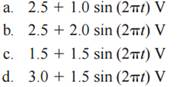### Create an Account

Already have account?

### Forgot Your Password ?

Home / Questions / Given a Schmitt trigger operating between 0 and 5 V assume that the lower threshold is 1 V...

# Given a Schmitt trigger operating between 0 and 5 V assume that the lower threshold is 1 V and the upper threshold is 4 V Given the following input signals applied to the Schmitt trigger sketch the

Given a Schmitt trigger operating between 0 and 5 V, assume that the lower threshold is 1 V and the upper threshold is 4 V. Given the following input signals applied to the Schmitt trigger, sketch the corresponding output from the Schmitt trigger for one cycle of each signal:Jul 26 2020 View more View Less

#### Answer (Solved)Subscribe To Get Solution